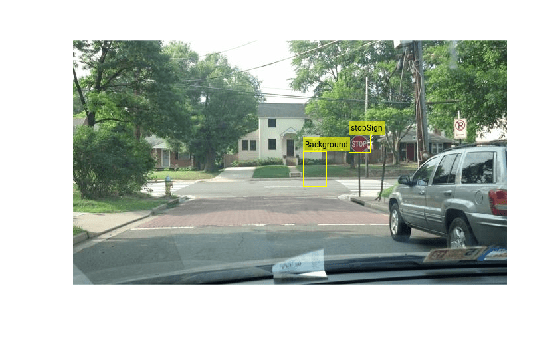# classifyRegions

Classify objects in image regions using Fast R-CNN object detector

## Syntax

``````[labels,scores] = classifyRegions(detector,I,rois)``````
``````[labels,scores,allScores] = classifyRegions(detector,I,rois)``````
``[___] = classifyRegions(___,'ExecutionEnvironment',resource)``

## Description

example

``````[labels,scores] = classifyRegions(detector,I,rois)``` classifies objects within the regions of interest of image `I`, using a Fast R-CNN (regions with convolutional neural networks) object detector. For each region, `classifyRegions` returns the class label with the corresponding highest classification score.When using this function, use of a CUDA® enabled NVIDIA® GPU is highly recommended. The GPU reduces computation time significantly. Usage of the GPU requires Parallel Computing Toolbox™. For information about the supported compute capabilities, see GPU Computing Requirements (Parallel Computing Toolbox).```
``````[labels,scores,allScores] = classifyRegions(detector,I,rois)``` also returns all the classification scores of each region. The scores are returned in an M-by-N matrix of M regions and N class labels.```
````[___] = classifyRegions(___,'ExecutionEnvironment',resource)` specifies the hardware resource used to classify object within image regions: `'auto'`, `'cpu'`, or `'gpu'`. You can use this syntax with either of the preceding syntaxes.```

## Examples

collapse all

Configure a Fast R-CNN object detector and use it to classify objects within multiple regions of an image.

Load a `fastRCNNObjectDetector` object that is pretrained to detect stop signs.

```data = load('rcnnStopSigns.mat','fastRCNN'); fastRCNN = data.fastRCNN;```

Read in a test image containing a stop sign.

```I = imread('stopSignTest.jpg'); figure imshow(I)```Specify regions of interest to classify within the test image.

```rois = [416 143 33 27 347 168 36 54];```

Classify the image regions and inspect the output labels and classification scores. The labels come from the `ClassNames` property of the detector.

`[labels,scores] = classifyRegions(fastRCNN,I,rois)`
```labels = 2x1 categorical stopSign Background ```
```scores = 2x1 single column vector 0.9969 1.0000 ```

The detector has high confidence in the classifications. Display the classified regions on the test image.

```detectedI = insertObjectAnnotation(I,'rectangle',rois,cellstr(labels)); figure imshow(detectedI)```## Input Arguments

collapse all

Fast R-CNN object detector, specified as a `fastRCNNObjectDetector` object. To create this object, call the `trainFastRCNNObjectDetector` function with training data as input.

Input image, specified as a real, nonsparse, grayscale or RGB image.

Data Types: `uint8` | `uint16` | `int16` | `double` | `single`

Regions of interest within the image, specified as an M-by-4 matrix defining M rectangular regions. Each row contains a four-element vector of the form [x y width height]. This vector specifies the upper left corner and size of a region in pixels.

Hardware resource used to classify image regions, specified as `'auto'`, `'gpu'`, or `'cpu'`.

• `'auto'` — Use a GPU if it is available. Otherwise, use the CPU.

• `'gpu'` — Use the GPU. To use a GPU, you must have Parallel Computing Toolbox and a CUDA enabled NVIDIA GPU. If a suitable GPU is not available, the function returns an error. For information about the supported compute capabilities, see GPU Computing Requirements (Parallel Computing Toolbox).

• `'cpu'` — Use the CPU.

Example: `'ExecutionEnvironment','cpu'`

## Output Arguments

collapse all

Classification labels of regions, returned as an M-by-1 categorical array. M is the number of regions of interest in `rois`. Each class name in `labels` corresponds to a classification score in `scores` and a region of interest in `rois`. `classifyRegions` obtains the class names from the input `detector`.

Highest classification score per region, returned as an M-by-1 vector of values in the range [0, 1]. M is the number of regions of interest in `rois`. Each classification score in `scores` corresponds to a class name in `labels` and a region of interest in `rois`. A higher score indicates higher confidence in the classification.

All classification scores per region, returned as an M-by-N matrix of values in the range [0, 1]. M is the number of regions in `rois`. N is the number of class names stored in the input `detector`. Each row of classification scores in `allscores` corresponds to a region of interest in `rois`. A higher score indicates higher confidence in the classification.

## Version History

Introduced in R2017a